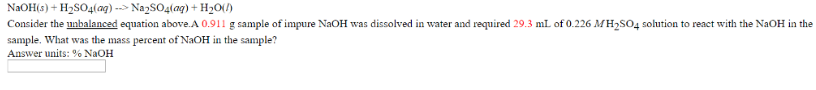# Problem: NaOH (s) + H2SO4 (aq) → Na2SO4 (aq) + H2O Consider the unbalanced equation above. A 0.911 g sample of impure NaOH was dissolved in water and required 29.3 mL of 0.226 M H2SO4 solution to react with the NaOH in the sample. What was the mass percent of NaOH in the sample?

###### FREE Expert Solution
94% (177 ratings)###### Problem Details

NaOH (s) + H2SO4 (aq) → Na2SO4 (aq) + H2

Consider the unbalanced equation above. A 0.911 g sample of impure NaOH was dissolved in water and required 29.3 mL of 0.226 M H2SO4 solution to react with the NaOH in the sample. What was the mass percent of NaOH in the sample?